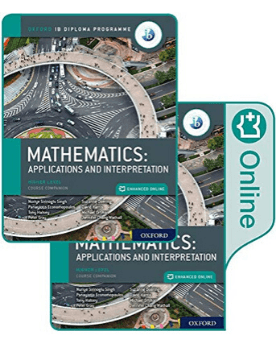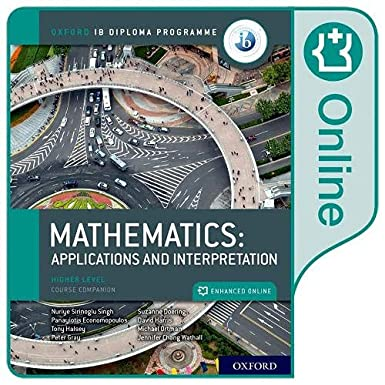# IB Mathematics: applications and interpretation HL Course Companion

Regular price \$89.00
Sale price \$89.00 Regular price \$115.00
Featuring a wealth of digital content, this concept-based Print and Enhanced Online Course Book Pack has been developed in cooperation with the IB to provide the most comprehensive support for the new DP Mathematics: applications and interpretation HL syllabus, for first teaching in September 2019.

### Features

• Address all aspects of the new DP Mathematics: applications and interpretation HL syllabus via an Enhanced Online Course Book Pack - made up of one full-colour, print textbook and one online textbook, including extensive teacher notes
• Ensure learners are ready to tackle each topic with targeted 'Prior Knowledge' worksheets, linked to 'Before You Start' summaries and exercises at the start of every chapter
• Deliver in-depth coverage of all topics through clear explanations and worked solutions, animated worked examples, differentiated exercises and worksheets, with answers provided
• Adopt a concept-based approach with conceptual lenses and microconcepts woven into every chapter, plus rich investigations that integrate factual and conceptual questions - leading to meaningful, content-specific conceptual understanding
• Deepen mathematical understanding via inquiry-based tasks that relate to the content of each chapter, 'international mindedness' features, regular links to Theory of Knowledge, and activities that target ATL skills
• Support students' development of a mathematical toolkit, as required by the new syllabus, with modelling and investigation activities presented in each chapter, including prompts for reflection, and suggestions for further study
• Thoroughly prepare students for IB assessment via in-depth coverage of course content, overviews of all requirements, exam-style practice questions and papers, and a full chapter supporting the new mathematical exploration (IA)
• Includes support for the most popular Graphic Display Calculator models
• This Online Course Book will be available on Oxford Education Bookshelf until 2029. Access is facilitated via a unique code, which is sent in the mail. The code must be linked to an email address, creating a user account

Measuring space: accuracy and geometry
1.1: Representing numbers exactly and approximately
1.2: Angles and triangles
1.3: three-dimensional geometry
Representing and describing data: descriptive statistics
2.1: Collecting and organizing data
2.2: Statistical measures
2.3: Ways in which we can present data
2.4: Bivariate data
Dividing up space: coordinate geometry, lines, Voronoi diagrams, vectors
3.1: Coordinate geometry in 2 and 3 dimensions
3.2: The equation of a straight line in 2 dimensions
3.3: Voronoi diagrams
3.4: Displacement vectors
3.5: The scalar and vector product
3.6: Vector equations of lines
Modelling constant rates of change: linear functions and regressions
4.1: Functions
4.2: Linear models
4.3: Inverse functions
4.4: Arithmetic sequences and series
4.5: Linear regression
Quantifying uncertainty: probability
5.1: Theoretical and experimental probability
5.2: Representing combined probabilities with diagrams
5.3: Representing combined probabilities with diagrams and formulae
5.4: Complete, concise and consistent representations
Modelling relationships with functions: power and polynomial functions
6.3: Cubic functions and models
6.4: Power functions, inverse variation and models
Modelling rates of change: exponential and logarithmic functions
7.1: Geometric sequences and series
7.2: Financial applications of geometric sequences and series
7.3: Exponential functions and models
7.4: Laws of exponents - laws of logarithms
7.5: Logistic models
Modelling periodic phenomena: trigonometric functions and complex numbers
8.1: Measuring angles
8.2: Sinusoidal models: f(x) = asin(b(x-c))+d
8.3: Completing our number system
8.4: A geometrical interpretation of complex numbers
8.5: Using complex numbers to understand periodic models

Modelling with matrices: storing and analyzing data
9.1: Introduction to matrices and matrix operations
9.2: Matrix multiplication and properties
9.3: Solving systems of equations using matrices
9.4: Transformations of the plane
9.5: Representing systems
9.7: Eigenvalues and eigenvectors
Analyzing rates of change: differential calculus
10.1: Limits and derivatives
10.2: Differentiation: further rules and techniques
10.3: Applications and higher derivatives
Approximating irregular spaces: integration and differential equations
11.1: Finding approximate areas for irregular regions
11.2: Indefinite integrals and techniques of integration
11.3: Applications of integration
11.4: Differential equations
11.5: Slope fields and differential equations
Modelling motion and change in 2D and 3D: vectors and differential equations
12.1: Vector quantities
12.2: Motion with variable velocity
12.3: Exact solutions of coupled differential equations
12.4: Approximate solutions to coupled linear equations
Representing multiple outcomes: random variables and probability distributions
13.1: Modelling random behaviour
13.2: Modelling the number of successes in a fixed number of trials
13.3: Modelling the number of successes in a fixed interval
13.4: Modelling measurements that are distributed randomly
13.5: Mean and variance of transformed or combined random variables
13.6: Distributions of combined random variables
Testing for validity: Spearman's hypothesis testing and x2 test for independence
14.1: Spearman's rank correlation coefficient
14.2: Hypothesis testing for the binomial probability, the Poisson mean and the product moment correlation coefficient
14.3: Testing for the mean of a normal distribution
14.4: Chi-squared test for independence
14.5: Chi-squared goodness-of-fit test
14.6: Choice, validity and interpretation of tests
Optimizing complex networks: graph theory
15.1: Constructing graphs
15.2: Graph theory for unweighted graphs
15.3: Graph theory for weighted graphs: the minimum spanning tree
15.4: Graph theory for weighted graphs - the Chinese postman problem
15.5: Graph theory for weighted graphs - the travelling salesman problem
Exploration

Author: Panayiotis Economopoulos, Author Tony Halsey, Author Suzanne Doering, Michael Ortman, Nuriye Sirinoglu Singh, Peter Gray, David Harris, and Jennifer Chang Wathall

Curriculum: IB Diploma Programme International Baccalaureate

Dimension: 10.1 x 1.3 x 7.7 inches

Format: Paperback

Isbn 10: 0198427042

Language: English

Pages: 856 pages

Release date: 05/15/2019

Series: Oxford IB Diploma Programme: IB Mathematics

Year: 2019